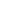# Free CBSE Class 9 Mathematics Unit 4-Geometry Circles Worksheets

Download free printable Circles Worksheets to practice. With thousands of questions available, you can generate as many Circles Worksheets as you want.

## Sample CBSE Class 9 Mathematics Unit 4-Geometry Circles Worksheet Questions

1.

In the figure, O is the centre of circle and AO is perpendicular to BO. Find .1. 2. 3. 4.
2.

In the given figure . Find .1. 2. 3. 4.
3.

Two concentric circles are with center O in the given figure. If AD = 10 cm and BC = 6 cm find the length of CD.1. 1 cm 2. 4 cm 3. 2 cm 4. 3 cm
4.

In the given figure, O is the centre of the circle. Find .1. 2. 3. 4.
5.

In the given figure BD = DC and . Find .1. 2. 3. 4.
6.

In the given figure, O is the centre of a circle and ABE is a straight line. Find x.1. 2. 3. 4.
7.

In the given figure, O is the centre of the circle. Find .1. 2. 3. 4.
8.

ABCD is a parallelogram, a circle through A,B,C intersect CD produced at E. Then  AD = AE. Is it True/False?1. True 2. False
9.

AB and CD are equal chords of a circle whose centre is O. When produced, these chords meet at E. Then EB = ED.1. True 2. False
10.

In the given figure, O is the centre of the circle, . Find  y.1. 2. 3. 4.

Worksheets by UrbanPro

Our worksheets are designed to help students explore various topics, practice skills and enrich their subject knowledge, to improve their academic performance. Designed by Experts who have extensive experience and expertise in teaching a subject, these worksheets will improve your child's problem-solving skills and subject knowledge in a fun and interactive manner.
Check out our free customized worksheets across school boards, grades, subjects and levels of subject knowledge. You can download, print and share these worksheets with anyone, anywhere, anytime!

Get a custom worksheet to practice!

Select your topic & see the magic.

subjectSelect Chapter(s)

Chapters & Subtopics